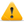Study the following formula:

3x2 + 5x3

Select the answer which represents this formula in its simplest terms:

The correct answer is 15x5

Don’t forget that you must add the indices 2 + 3 = 5

Then perform the usual algebraic multiplicationThis is a sample question. You can start the practice exam by clicking the 'Start Practice Exam' link on the exam page.

Back to practice exam

Free practice exams are provided free of charge by Accelerated Ideas. Please respect our exams and do not reproduce our material or abuse the testing system. Thank you. Maths practice tests for SAT Maths and GCSE Maths
Home
Copyright © Accelerated Ideas 2005-2019
All rights reserved